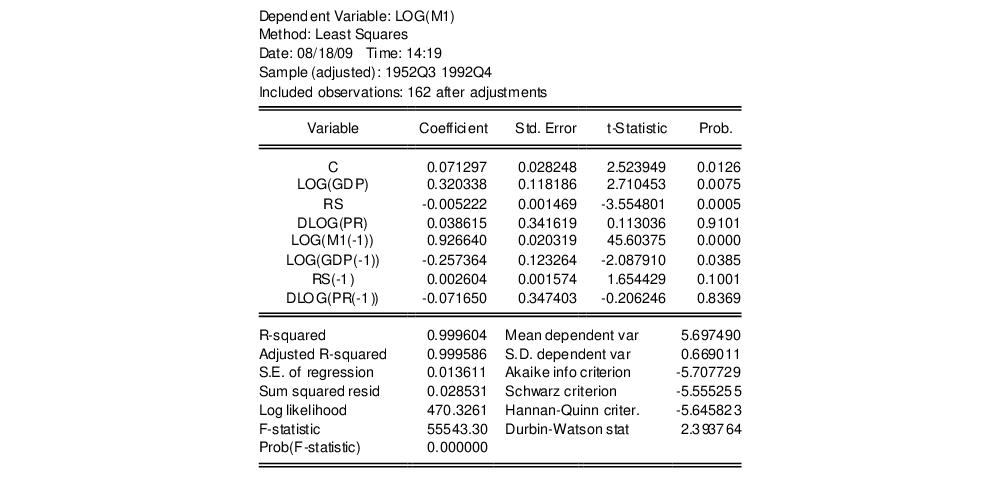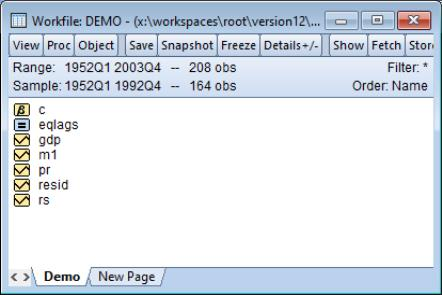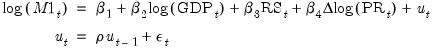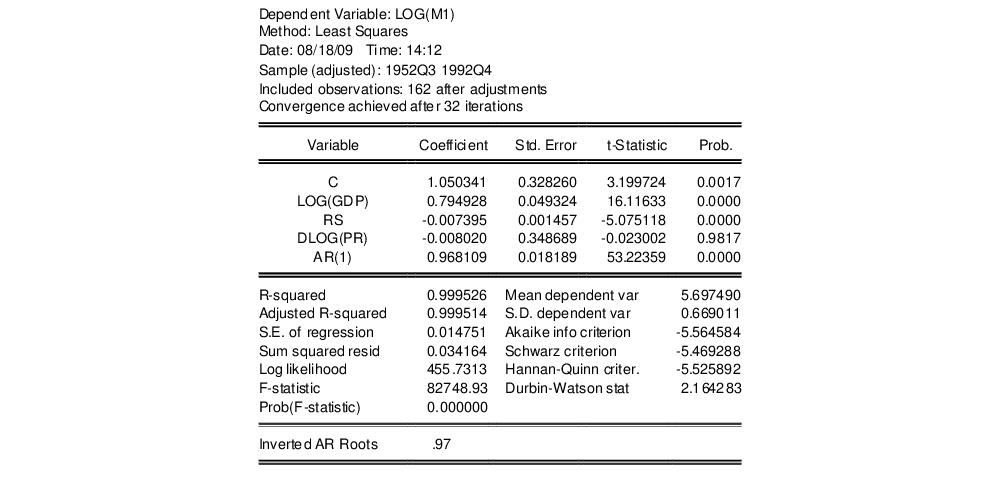User’s Guide : EViews Fundamentals : A Demonstration : Modifying the Equation

Modifying the Equation
The test results suggest that we need to modify our original specification to take account of the serial correlation.
One approach is to include lags of the independent variables. To add variables to the existing equation, click on the Estimate button in the equation toolbar and edit the specification to include lags for each of the original explanatory variables:
log(m1) c log(gdp) rs dlog(pr) log(m1(-1)) log(gdp(-1)) rs(-1) dlog(pr(-1))
Note that lags are specified by including a negative number, enclosed in parentheses, following the series name. Click on OK to estimate the new specification and to display the results:Note that EViews has automatically adjusted the estimation sample to accommodate the additional lagged variables. We will save this equation in the workfile for later use. Press the Name button in the toolbar and name the equation EQLAGS.The EQLAGS equation object will be placed in the workfile.
One common method of accounting for serial correlation is to include autoregressive (AR) and/or moving average (MA) terms in the equation. To estimate the model with an AR(1) error specification, you should make a copy of the EQLAGS equation by clicking Object/Copy Object… in the EQLAGS window. EViews will create a new untitled equation containing all of the information from the previous equation. Press Estimate on the toolbar of the copy and modify the specification to read
log(m1) c log(gdp) rs dlog(pr) ar(1)
This specification removes the lagged terms, replacing them with an AR(1) specification:(1.2)
Click OK to accept the new specification. EViews will estimate the equation and will report the estimation results, including the estimated first-order autoregressive coefficient of the error term:The fit of the AR(1) model is roughly comparable to the lag model, but its somewhat higher values for both the Akaike and the Schwarz information criteria indicate that the previous lag model may be preferred. Accordingly, we will work with the lag model in EQLAGS for the remainder of the demonstration.